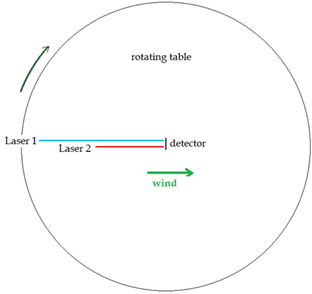Two Laser One-Way Velocity of Light InterferometerArm 1 Length[ L] Refractive Index Arm 1 c' (with wind): c" (Against Wind):
Arm 2 Length [L] Refractive Index Arm 2
aether wind [V] (m/s) light l (m) l' (into wind): l" (against wind):
Lorentz Contraction [1/g]: Freq. @ 632.8nm

Fringe Shift Due to Path Effect: Fringe Shift Due to Clock Effect:
Method 1: Laser 1 Laser 2
Angle to Wind: Arm 1 forward Time Dt Arm1-Arm2 (s) Fringe Shift: [t] (s) [t] (s)
0 4p*(L/l)*(v/c)
0 Fringe Difference: [sin(q)] for 180 deg. [sin(q)] for 180 deg.
Arm 2 forward Time
Arm 1 forward Dt Arm1-Arm2 (s) Time Difference: sin(q)/2 for 90 deg. sin(q)/2 for 90 deg.
180
180 Fringe Difference   Velocity of Table m/s Velocity of Table m/s
Arm 2 forward
Method 2: angular velocity [wrot] Laser 1 angular velocity [wrot] Laser 2
Angle to wind: Phase at laser1 c*t/l Phase at Det. f*t+L1/gl' Fringe Shift:
0 4p*(L/l)*(v/c) radius Laser 1 [L] (m) radius Laser 2 [L] (m)
Phase at laser2 c*t/l Phase at Det. f*t+L1/gl'
0   Vspin Laser 1 (m/s) Vspin Laser 2 (m/s)
Phase at laser1 c*t/l Phase at Det. f*t+L1/gl'' Time Difference:
180 Dt on Laser 1 (s) Dt on Laser 2 (s)
Phase at laser2 c*t/l Phase at Det. f*t+L1/gl''   t*(1/(1-v^2/c^2)*(L/2c^2)*V*wrot*sin(q) t*(1/(1-v^2/c^2)*(L/2c^2)*V*wrot*sin(q)
180
fringe shift on laser 1 due to Df fringe shift on laser 2 due to Df
Measurement t (s): Distance between lasers (m) Change in Phase 0-180: Final Dfringe: t*(1/(1-v^2/c^2)*(L/2cl)*V*wrot*sin(q) t*(1/(1-v^2/c^2)*(L/2cl)*V*wrot*sin(q)
Path-Clock Effect:
Change in Phase 0-180: Dfringe laser1 - laser2: Dt Laser1 - Laser2

Why Can't a One-Way Velocity of Light Experiment Detect an Aether Drift even if the Aether Exists?
One-way velocity of light experiments fail to detect an aether drift since any fringe shift due to a difference in the one way velocity of light is cancelled by a shift in the clock frequency of the laser. This example describes what happens when two synchronized lasers are at different distances from the same detector, where their respective beams interfere. If the table is rotated so the lasers go from parallel to perpendicular to the aether wind (rotated 180 degrees), a fringe shift should arise due to the path length differences and the changing speed of light in different directions. However, this fringe shift is exactly cancelled by a change in the clock frequency of each laser, due to a mechanical time dilation effect predicted by Lorentz. This is regardless of the position of each laser on the table. Different positions can be simulated by changing the arm lengths for each laser above. A negative length value switches the laser to the right side of the table in the diagram. Green values are adjustable -the refractive index of the arms and the table edge rotation velocity can also be altered, as can the wavelength of the laser light and the velocity of the aether wind. Hit update for the new values to take effect. Two different methods are used to calcualte the path fringe shift - method 1 uses the propagation time along each arm; method 2 counts the wavelengths along each arm at the measurement time, yielding the same result. To avoid errors, a small value should be used for the measurement time, which is distinct from the rotation time [t] used in the laser clock effect calculation.